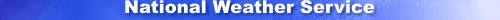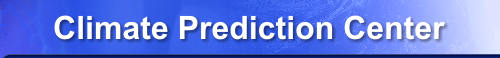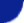Site Map News Organization SearchHOME > Monitoring_and_Data > Oceanic and Atmospheric Data > Reanalysis: Atmospheric Data > wgrib2-import_netcdf

### wgrib2: -import_netcdf (alpha)

The -import_netcdf option is alpha code which means that the syntax is bound to change. You've been warned.

My second use of this option (wgrib2 v3.0.0) improved the code. There were bug fixes and the ability to import values stored in bytes and integers. The second use converted the OSTIA SST files from netcdf to grib. The grib2 file was 8% of the original size. (92% size reduction). The grib2 compression was extremely good, using only 0.97 bits to store each grid point value. The grib compression was good because one field was a mask, ice concentration was mostly zero value, and the SST and SST error where undefined over land and lakes. The grid point values were unchanged in the conversion from netcdf to grib because both the netcdf and grib files used the same precision.

### Introduction

Reading netcdf is easy if someone else wrote the program to read the netcdf file. Writing the code to read netcdf is something else. The -import_netcdf option allows you to read/import arbitrary netcdf variables into wgrib2.

A netcdf file can contain many variables. In my sample file, there is one data varable "acpcp" and and 6 supporting variables. variables are

```    variable 0: time
variable 1: lat(x,y)                 latitude
variable 2: lon(x,y)                 longitude
variable 3: y                        integer
variable 4: x                        integer
variable 5: Lambert_Conformal        grid definition
variable 6: acpcp(x,y,time)          accumulated convective precip
```

Netcdf variables have dimensions which can be of arbitrary units. For example the time is not stored as YYYYMMDDHHSS but can be stored as "nanoseconds since 1981-2-23", "fortnights since 1990-11-25 12:00 EST" or anything else you can imagine. Geopotential height can have units of meters, metres, inches, microns, nautical miles, chains, rods or any unit of length in any language (necdf supports unicode). The joys of standards that don't set standards. (Netcdf is a standard but fails to standardize units of length.)

Variables in netcdf can be viewed as variable(dim-i,dim-j,..,dim-last) where the number of dimensions differ. For example, in one dataset, X could be one-dimensional, latitude could be two dimensional and temperature could be 4 dimensional.

There is netcdf library code to read varaible(i0:i1, j0:j1, .. , last0:last1). With -import_netcdf, you specifiy the netcdf file, the netcdf variable and "i0:i1,..,last0:last1". The code reads the data and uses it to overwrite the wgrib2's data register. For this option, you don't specify "i0:i1" but "i0" and "n", the number of to read (i1=i0+n-1).

### Tutorial: Variables in an unknown netcdf file

In this example, I am trying to read a NARR netcdf file produced by a 3rd party. As a first step, I want to list the variables in the netcdf file.

```acpcp.1979.nc = netcdf file

small.grb2 = arbitary grib2 file (used so that -import_netcdf will run)
asd = junk name that doesn't match a variable name or dimension specification

sh-4.1\$ wgrib2  ~/grib2/examples/small.grb2 -import_netcdf acpcp.1979.nc asd asd
import_netcdf: asd is not valid variable, valid variables are
variable 0: time
variable 1: lat
variable 2: lon
variable 3: y
variable 4: x
variable 5: Lambert_Conformal
variable 6: acpcp

In the above line, I am importing the variable "asd" from netcdf file "apcpc.1979.nc".
As expected, "asd" is not a valid varaible, and I get an error message and the
desired list of valid variables.
```

### Tutorial: Unknown attributes and dimensions of a variable

To get a variable description, you need to try to import a netcdf file. acpcp.1979.nc with a valid variable, acpcp, with an invalid dimension specification, "asd".
```bash-4.1\$ wgrib2 ~/grib2/examples/small.grb2 -import_netcdf acpcp.1979.nc acpcp asd
ndims=3 var_type=5 #var_attributes 16
acpcp.0 attr=GRIB_id type=3 len=1
acpcp.1 attr=GRIB_name: ACPCP
acpcp.2 attr=coordinates: lat lon
acpcp.3 attr=dataset: NARR 3-hourly
acpcp.4 attr=grid_mapping: Lambert_Conformal
acpcp.5 attr=level_desc: Surface
acpcp.6 attr=long_name: 3-hourly accumulated convective precipitation at Surface
acpcp.7 attr=parent_stat: Other
acpcp.8 attr=standard_name: convective_precipitation_amount
acpcp.9 attr=statistic: Individual Obs
acpcp.10 attr=units: kg/m^2
acpcp.11 attr=valid_range type=5 len=2
acpcp.12 attr=var_desc: convective precipitation accumulation
acpcp.13 attr=missing_value type=5 len=1
acpcp.14 attr=actual_range type=5 len=2
acpcp.15 attr=_FillValue type=5 len=1
_FillValue=9969209968386869046778552952102584320.000000 1 missing_value=-9969209968386869046778552952102584320.000000 1
dimension mismatch asd, netcdf file has
dim 0 id=0 name=time recs=2920
dim 1 id=1 name=y recs=277
dim 2 id=2 name=x recs=349

*** FATAL ERROR: import_netcdf: dimensions do not match ***
```
By using an invalid dimension, the attributes and dimensions of the variable are listed. From the attributes, we see that the variable is a 3 hour accumulated convective precipitation. (Don't know whether it is the 0-3 hour accumulated forecast or n-n+3 hour hour forecast.)

Wgrib2 is using the C interface to the netcdf library. So C conventions apply. For example, indices start at zero such as the dimension number.

The dimension description shows that acpcp is is a 3 dimensional variable, apcpcp(time, y, x).

### Tutorial: Grid of an unknown netcdf file

We can get the description of the latitude by
```1:0bash-4.1\$ wgrib2 ~/grib2/examples/small.grb2 -import_netcdf acpcp.1979.nc lat asd
ndims=2 var_type=5 #var_attributes 5
lat.0 attr=axis: Y
lat.1 attr=coordinate_defines: point
lat.2 attr=long_name: Latitude
lat.3 attr=standard_name: latitude
lat.4 attr=units: degrees_north
_FillValue=0.000000 0 missing_value=0.000000 0
dimension mismatch asd, netcdf file has
dim 0 id=1 name=y recs=277
dim 1 id=2 name=x recs=349

*** FATAL ERROR: import_netcdf: dimensions do not match ***
```
Similarily we can find the description of lon. We see that lat(y,x) and lon(y, x).

### Tutorial: Projection of an unknown netcdf file

The NARR grid is a Lambert Conformal grid. The grid description is stored in the Lambert_Conformal variable.
```bash-4.1\$ wgrib2 ~/grib2/examples/small.grb2 -import_netcdf acpcp.1979.nc Lambert_Conformal asd
ndims=0 var_type=4 #var_attributes 6
Lambert_Conformal.0 attr=false_easting type=6 len=1
Lambert_Conformal.1 attr=false_northing type=6 len=1
Lambert_Conformal.2 attr=grid_mapping_name: lambert_conformal_conic
Lambert_Conformal.3 attr=latitude_of_projection_origin type=6 len=1
Lambert_Conformal.4 attr=longitude_of_central_meridian type=6 len=1
Lambert_Conformal.5 attr=standard_parallel type=6 len=2
_FillValue=0.000000 0 missing_value=0.000000 0
WARNING: import_netcdf: size mismatch grib:4 netcdf:1
1:0:d=2009060500:HGT:200 mb:180 hour fcst:ENS=+19
```

### Tutorial: converting a netcdf field to grib2

In our example, we have a NARR grid and we can find NARR grib1 files online from NCEI. Using grb1to2.pl, we can convert a NARR file into grib2 and make a sample grib2 file. This template will use the same grid as our netcdf file. The following will read the 1st field from acpcp.1979.nc and convert it into grib2.
```bash-4.1\$ wgrib2 narr.grb2 -import_netcdf acpcp.1979.nc acpcp "0:1:0:277:0:349" -set_var ACPCP \
-set_lev surface -set_date 1979010100 -set_ftime2 "0-3 hour acc fcst" -grib_out out.grb2
ndims=3 var_type=5 #var_attributes 16
acpcp.0 attr=GRIB_id type=3 len=1
acpcp.1 attr=GRIB_name: ACPCP
acpcp.2 attr=coordinates: lat lon
acpcp.3 attr=dataset: NARR 3-hourly
acpcp.4 attr=grid_mapping: Lambert_Conformal
acpcp.5 attr=level_desc: Surface
acpcp.6 attr=long_name: 3-hourly accumulated convective precipitation at Surface
acpcp.7 attr=parent_stat: Other
acpcp.8 attr=standard_name: convective_precipitation_amount
acpcp.9 attr=statistic: Individual Obs
acpcp.10 attr=units: kg/m^2
acpcp.11 attr=valid_range type=5 len=2
acpcp.12 attr=var_desc: convective precipitation accumulation
acpcp.13 attr=missing_value type=5 len=1
acpcp.14 attr=actual_range type=5 len=2
acpcp.15 attr=_FillValue type=5 len=1
_FillValue=9969209968386869046778552952102584320.000000 1 missing_value=-9969209968386869046778552952102584320.000000 1
1:0:d=1979010100:ACPCP:surface:0-3 hour acc fcst:

-import_netcdf acpcp.1979.nc acpcp "0:1:0:277:0:349"
0:1:0:277:349 = hyperslab specifier
0:1   for the 1st dimension (t), start at 0 and loop 1 time
(if your file has multiple times, to get the second
time, you use "1:1".)
0:277 for the 2nd dimension (y), start at 0 and loop 277 times
0:349 for the 3rd dimension (x), start at 0 and loop 349 times
-set_var ACPCP                   change the grib variable name to ACPCP of the memory buffer
-set_lev surface                 change the grib level to surface of the memory buffer
-set_date 1979010100             change the reference data of the memory buffer
-set_ftime2 "0-3 hour acc fcst"  change the time stamp of the memory buffer
-grib_out out.grb2               write memory buffer to out.grb2

-import_netcdf reads a hyberslab of dimension 1 x 277 x 349.  Now the narr.grb2 has
a grid of 349 x 277.  You can check that the grib2 scan order is compatible with
the netcdf scan order either by examining the lat/lon or by checking the netcdf
file with the grib file obtained from another source.
```

The -import_netcdf option is not convenient and takes some work to setup. However, it did work and I was able to verify that the netcdf NARR file was the same as my grib copy. For lat-lon netcdf files that follow the coards convention, using GrADS and the g2grb.gs script is easier to use.

### Warnings

The -import_netcdf option does very little error checking. Suppose your netcdf hyper-cube is organized WE:NS, and your in-memory grid is WE:SN (default). The -import_netcdf directly over-write the in-memory grid with the netcdf hyper-cube. The user is responsible it reordering the data (-rpn -yrev). As of 3/2022, only WE:NS can be transformed to WE:SN.

### Usage

```-import_netcdf file variable "dim_list"
file     = netcdf file
variable = variable in the netcdf file
dim_list    = specification of the hyperslab to read
dim_list    = dim_spec:dim_spec:..:dim_spec
dim_spec    = dim_start:dim_loop
dim_start   = index for start of hyberslap starting at 0
dim_loop    = number time to loop
```

### More Examples

See Converting OSTIA SST in netcdf to grib.